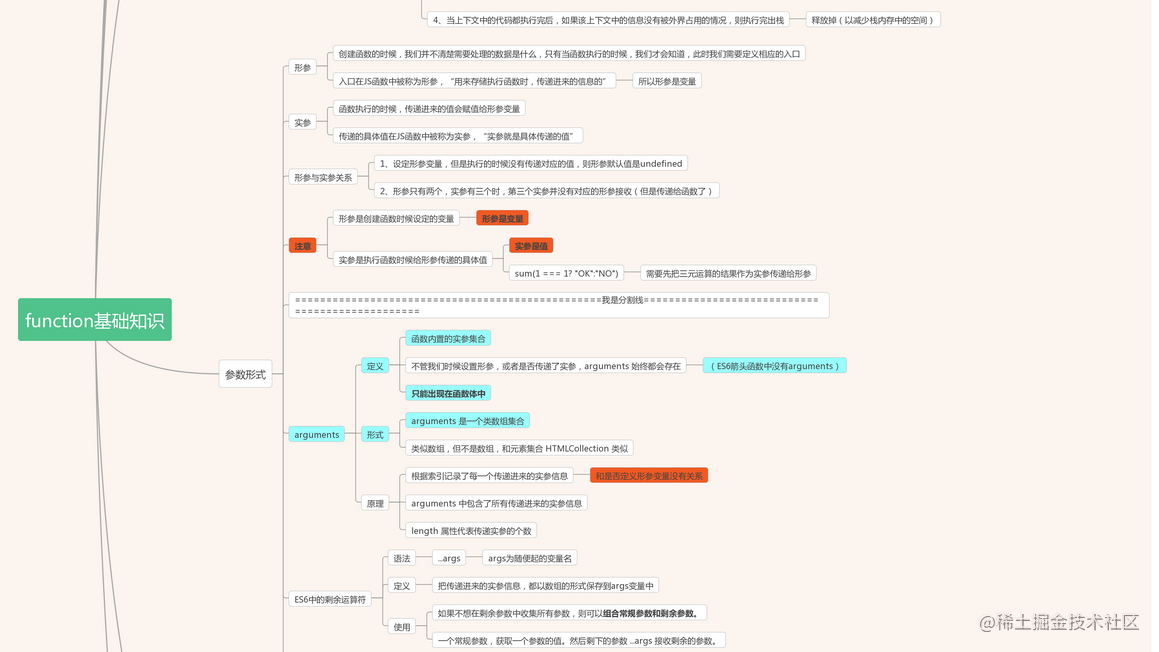# 一、什么是函数

## 1、实质与意义

• 函数实际上就是一种方法；意义在于封装

## 2、应用：

• 把实现某一个功能的代码封装在一起，后期想实现这个功能，只需要执行函数即可，不需要重新编写这些代码了

## 3、目的：

• 减少页面中冗余代码，提高代码重复利用率，“低耦合，高内聚”

# 二、函数的两个重要部分

## 1、创建函数

### -1).语法：

• function 函数名(形参){ 函数体 }

### -2).创建过程：

• 第一步：
• 创建值：
• 1、开辟一个堆内存
• 2、把函数体中的代码当作字符串储存在堆中
• 3、把堆地址放到栈中
• 第二步：
• 创建变量
• 第三步：
• 让变量和地址关联

## 2、执行函数

• 函数名（实参）

### -2).目的

• 把创建的函数执行（把函数体中的代码执行）

• 栈内存
• 供代码执行的上下文

### -4).执行过程：

• 第一步：
• 创建一个全新的执行上下文，把执行上下文压缩到栈内存中去执行（进栈执行）
• 第二步：
• 在这个上下文中，也存在一个AO（变量对象），用来存储当前上下文代码执行中所创建的变量
• 这些变量是“私有变量”
• 除当前上下文中可以使用这些变量的值，上下文以外的环境不能直接使用私有变量的值
• ......先讲解基础知识，所以我们中间省略了一些细化的步骤，后续会一点一点完善；
• 第三步：
• 代码执行
• 第四步：
• 当上下文中的代码都执行完后，如果该上下文中的信息没有被外界占用的情况，则执行完出栈（释放掉）以减少栈内存中的空间；

# 三、参数的形式

## 1、形参

• 定义：
• 创建函数的时候，我们并不清楚需要处理的数据是什么，只有当函数执行的时候，我们才会知道，此时我们需要定义相应的入口，这个入口在JS函数中被称为形参；
• 作用：
• “用来存储执行函数时，传递进来的信息”
• 所以形参是变量

## 2、实参

• 定义：
• 函数执行的时候，传递进来的值会赋值给形参变量，传递的具体值在JS函数中被称为实参；
• 作用：
• “实参就是具体传递的值”
``````function sum(x, y) {
let total = x + y;
console.log(total);
}
sum(1 === 1 ? 'OK' : 'NO'); //=>也需要把三元表达式运算的结果作为实参传递给形参

let age = 20;
sum(age); //=> x=20  实参永远都应该是值，此处虽然写的AGE，但是他会把AGE变量的值拿到，然后传递给形参x，而不是把AGE本身传递过去   =>sum(20)

### 形参与实参的对应关系

• 1、设定形参变量，但是执行的时候没有传递对应的值，则形参默认值是`undefined`
• 2、形参只有两个，实参有三个时，第三个实参并没有对应的形参接收（但是传递给函数了）

## 3、arguments

• 定义：
• 函数内置的实参集合
• 不管我们时候设置形参，或者是否传递了实参，`arguments` 始终都会存在（`ES6`箭头函数中没有`arguments`
• 只能出现在函数体中
• 形式：
• `arguments` 是一个类数组集合
• 类似数组，但不是数组，和元素集合 `HTMLCollection` 类似
• 原理:
• 根据索引记录了每一个传递进来的实参信息 (和是否定义形参变量没有关系)
• `arguments`中包含了所有传递进来的实参信息
• `length` 属性代表传递实参的个数
• 栗子🌰： 任意数求和：不管传递几个实参值进来，我们都能求出对应的和
``````//=================任意数求和：不管传递几个实参值进来，我们都能求出对应的和
// 有一个具体的问题：基于形参变量来接收求和的数字已经不现实了，因为我们也不知道要传递多少个值，也就无法确定要定义多少个形参变量了
function sum() {
//  循环ARGUMENTS中的每一项（每一个传递进来的实参），都累加给TOTAL，最后就能求出和了
let total = 0;
for (let i = 0; i < arguments.length; i++) {
// 把传递进来的实参信息都转换为数字：排除掉非有效数字或者字符串
let item = Number(arguments[i]);
if (!isNaN(item)) {
// 有效数字
total += item;
}
}
console.log(total);
}
sum(); //=>0
sum(10); //=>10
sum(10, 20); //=>30
sum(10, 20, 30, 40); //=>100
sum(10, 20, 'AA'); //=>30 过滤掉非有效的数字
sum(10, '20'); //=>30 如果是字符串，不能是字符串拼接，还应该是数学相加

## 4、ES6中的剩余运算符

• 语法：
• `...args``args`为随便起的变量名）
• 定义：
• 把传递进来的实参信息，都以数组的形式保存到`args`变量中
• 使用：
• 如果不想在剩余参数中收集所有参数，则可以组合常规参数和剩余参数。
• 一个常规参数，获取一个参数的值。然后剩下的参数 `...args` 接收剩余的参数。
• 上面的🌰用剩余运算符实现：
``````function sum(...args) {
// ...args：ES6中的剩余运算符，把传递进来的实参信息，都已数组的形式保存到ARGS变量中
let total = 0;
for (let i = 0; i < args.length; i++) {
let item = Number(args[i]);
if (!isNaN(item)) {
total += item;
}
}
console.log(total);
}
sum(10, 20, 30, 40);

### 剩余参数和 arguments对象的区别

• 1、剩余参数只包含那些没有对应形参的实参，而 `arguments` 对象包含了传给函数的所有实参。
• 2、`arguments`对象不是一个真正的数组，而剩余参数是真正的 `Array`实例

# 四、返回值 return

• 定义：
• 那么，如果，外面想用当前上下文中的一些私有信息，则需要函数提供对应的出口，把信息提供给外面使用，而这个出口在`JS`函数中被称为“返回值 `return`
• 作用：
• 1、基于 `return` 把变量的值暴露给外面使用
• 在外边创建一个变量，用来接收函数执行返回的值（也就是 `return` 后面的值）
• 2、告知函数体中下面代码不在执行

return 后面放的一定是变量

• 例如:
• console.log（sum）:
• 这里输出的是 sum 函数本身;
• sum = 函数
• console,log (sum());
• 代表让函数执行
• 这里输出的是函数的返回值
• 如果函数中没有写`return`，默认的返回值是`undefined`

# 五、函数的表达形式

• 有函数名的

## 2、匿名函数

• -1).函数表达式
• 把一个函数当作值赋值给变量
• 事件绑定
``````document.body.onclick = function () {
// 此处就是匿名函数
};
let fn = function () {
// 也是匿名函数 fn代表这个函数 和 function fn类似
};
复制代码``````
• -2).自执行函数
• 函数创建完就立即执行了
• 创建函数时，`function`包一个小括号或前面加特殊字符是为了符合语法规范
• (function（n）{...})(实参):
• + function（n）{...}(实参)
• - function（n）{...}(实参)
• ~ function（n）{...}(实参)
• ! function（n）{...}(实参)

## 3、箭头函数

• 语法：
• let func = (x,y) => {...};
``````//==============箭头函数（ES6中新定义的创建函数的方式）
let func = (x, y) => {
// x和y是形参变量
// 大括号中还是函数体
};
func(10, 20);

//=>箭头函数可以简化函数编写的方式：如果函数体中只有一句RETURN，则可以忽略大括号和RETURN
let sum = (x, y) => x + y;

function sum(x, y) {
return x + y;
}

function func(x) {
return function (y) {
return x + y;
}
}
let func = x => y => x + y;